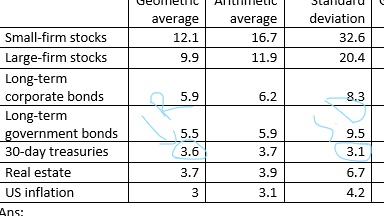# Standard Deviation of a risk free asset

In the portfolio management section, it is stated that a risk free asset has zero standard deviation and zero correlation of returns with those of a risky portfolio. I could be mistaken but I remember learning that 90 day US T-Bills are considered “risk-free assets” since in theory the US government will not default on these short term obligations therefore “in theory” they should have a standard deviation of returns of 0. Is this true? I believe I may have encountered a few practice problems where there was in fact a standard deviation of returns given for 90 day T-Bills which contradicts the “zero standard deviation” principle of a risk-free asset as explained in the portfolio management section.

Three-month T-bills are an approximation to risk-free assets; they’re not, in fact, risk-free.

The theoretical (i.e., nonexistent) risk-free asset has a zero standard deviation of returns, something that does not happen in the real world.

Got it, thanks. On the exam if there was a question along the lines of a “risk free treasury” such as the 90 day T-bill, should I assume the “real world” in that it probably does have a standard deviation of return, or go with the “theoretical” example and assume it does not? I don’t want to get tripped up by a technicality.

The exam won’t cite a “risk-free Treasury”. It will cite the risk-free asset.

OK - so when there is wording surrounding “the risk-free asset”, I should go ahead and assume the properties of a truly risk-free asset such as no standard deviation of returns even though no such as asset exists in the real world?

Yup.

if risk free asset has standard deviation like t-bill rate 2.6 and its S.D 1.5.what does it mean???

It means that it’s not truly risk-free.

how can i calculate risk premium of this aasest??

If you were to start with, say, a 13-week T-bill and a 52-week T-bill, you could use their expected returns and standard deviations of returns and extrapolate (linearly) to the expected return when the standard deviation of returns is zero.

Once you have that, the risk premium on any asset will be its expected return less the return you extrapolated for the zero-σ asset.actually this happend.

can u show me the equation??

It’s algebra I:

\frac{r_0\ -\ r_{52}}{σ_0\ -\ σ_{52}}\ =\ \frac{r_{13}\ -\ r_{52}}{σ_{13}\ -\ σ_{52}}
r_0\ -\ r_{52}\ =\ \left(\frac{r_{13}\ -\ r_{52}}{σ_{13}\ -\ σ_{52}}\right)\left(σ_0\ -\ σ_{52}\right)
r_0\ =\ r_{52}\ +\ \left(\frac{r_{13}\ -\ r_{52}}{σ_{13}\ -\ σ_{52}}\right)\left(σ_0\ -\ σ_{52}\right)